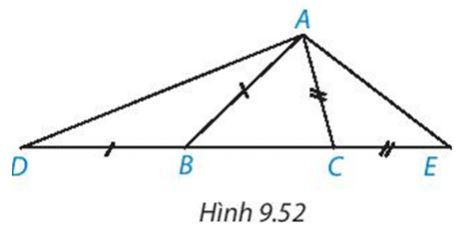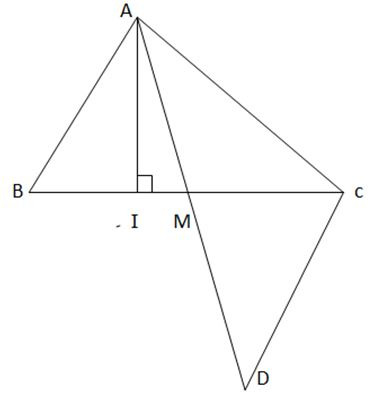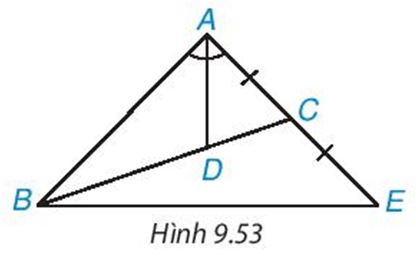## Solve the last exercise Chapter 9 Math 7 Connect – Math Book

Solve the last exercise Chapter 9 Math 7 Connect

==========

### Solve lesson 9.36 page 84 Math 7 textbook Connecting knowledge volume 2 – KNTT

Let ABC be a triangle $$\widehat{BAC}$$ an obtuse angle. Take point D between A and B, take point E between A and C (H.9.51). Prove DE < BC.

Detailed instructions for solving problem 9.36

Solution method

DC>DE (relation between angle and opposite side in triangle DEC). (first)

Consider triangle ADC => $$\widehat{BDC}$$ is an obtuse angle (2)

From (1) and (2) infer: BC>DE

Detailed explanation

Since $$\widehat{BAC}$$ is an obtuse angle, $$\widehat{ADE}$$ , $$\widehat{AED}$$ are acute angles

=> $$\widehat{DEC}$$ is an obtuse angle.

=>DC>DE (relation between angle and opposite side in triangle DEC). (first)

$$\widehat{DAC}$$ is an obtuse angle so $$\widehat{ADC}$$ , $$\widehat{ACD}$$ are acute angles

=> $$\widehat{BDC}$$ is an obtuse angle.

=>BC>DC (relationship between angle and opposite side in triangle BDC) (2)

– From (1) and (2) infer: BC>DE

–>

— *****

### Solve problem 9.37 page 84 Math textbook 7 Connecting knowledge volume 2 – KNTT

Let ABC be a triangle (AB > AC). On the line containing side BC, take point D and point E such that B lies between BD and C, C lies between B and E, BD = BA, CE = CA (H.9.52)

a) Compare $$\widehat{ADE}$$ and $$\widehat{AED}$$

b) Compare the line segments AD and AEDetailed instructions for solving problem 9.37

Solution method

a) AB > AC => $$\widehat{ABC}$$ < $$\widehat{ACB}$$, find the measure $$\widehat{ACE}$$, $$\widehat{ABD}$$ then compare

Triangle ABD is isosceles at B ( BD= BA) => $$\widehat{ABD}$$ = 180°- 2$$\widehat{ADB}$$

Triangle ACE is isosceles at C ( CE= CA) => $$\widehat{ACE}$$ = 180°- 2$$\widehat{AEC}$$

Find the measure of two angles $$\widehat{ADB}$$, $$\widehat{AEC}$$ and compare

Detailed explanation

a) AB > AC => $$\widehat{ABC}$$ < $$\widehat{ACB}$$ (relation between angle and opposite side in triangle ABC)

$$\widehat{ABD}$$ + $$\widehat{ABC}$$ = 180° => $$\widehat{ABC}$$ = 180°- $$\widehat{ABD}$$

$$\widehat{ACE}$$ + $$\widehat{ACB}$$ = 180° => $$\widehat{ACB}$$ = 180°- $$\widehat{ACE}$$

=> 180°- $$\widehat{ABD}$$ < 180°- $$\widehat{ACE}$$

=> $$\widehat{ACE}$$ < $$\widehat{ABD}$$

Triangle ABD is isosceles at B ( BD= BA) => $$\widehat{ABD}$$ = 180°- 2$$\widehat{ADB}$$

Triangle ACE is isosceles at C ( CE= CA) => $$\widehat{ACE}$$ = 180°- 2$$\widehat{AEC}$$

=> 180°- 2$$\widehat{ADB}$$ > 180°- 2$$\widehat{AEC}$$

=> $$\widehat{ADB}$$ < $$\widehat{AEC}$$

b) Considering triangle ADE, we have: $$\widehat{ADB}$$ < $$\widehat{AEC}$$

–>

— *****

### Solve problem 9.38 page 84 Math 7 textbook Connecting knowledge volume 2 – KNTT

Let AI and AM be the altitude and median, respectively, from the vertex A of triangle ABC. Prove that

a) AI < $$\frac{1}{2}$$ (AB + AC)

b) AM < $$\frac{1}{2}$$ (AB + AC)

Detailed instructions for solving problem 9.38

Solution method

a) AI is the altitude from A to the line segment BC

=> AI < AB and AI < AC

Adding the two sides together we have => AI <$$\frac{1}{2}$$ (AB + AC)

b) Take D such that M is the midpoint of AD

Considering ABM and DCM, prove that ABM = DCM

Detailed explanationa) AI is the altitude from A to the line segment BC=> AI is the distance from A to BC => AI is the shortest

=> AI < AB and AI < AC

Adding the two sides together we have: 2 AI < AB + AC

=> AI <$$\frac{1}{2}$$ (AB + AC)

b) Take D such that M is the midpoint of AD

Consider ABM and DCM have

AM = DM (M is mid point of AD)

BM=CM (M is mid point of BC)

$$\widehat{AMB}$$ = $$\widehat{CMD}$$ (2 opposite angles)

=> ABM = DCM

=> AB = CD

=> 2AM < AC + AB

=> AM < $$\frac{1}{2}$$ (AB + AC)

–>

— *****

### Solve problem 9.39 page 84 Math 7 textbook Connecting knowledge volume 2 – KNTT

Let ABC be a triangle with bisectors AD and D lying on BC such that BD = 2 DC. On the line AC, take point E such that C is the midpoint of AE (H.9.53). Prove that triangle ABE is isosceles at A

Hint D is the centroid of the triangle ABE, which has the bisector AD and the median.Detailed instructions for solving problem 9.39

Solution method

D is the centroid of triangle ABE, which has the bisector AD and is the median.

Detailed explanation

C is the midpoint of AE => BC is the median of triangle ABE (1)

D belongs to BC, BD= 2DC

=> BC= BD + DC = 2DC + DC = 3DC => DC = $$\frac{1}{3}$$ BC (2)

From (1) and (2)=> D is the centroid of triangle ABE

=> AD is the median line with BE

where AD is the bisector of $$\widehat{BAC}$$ or $$\widehat{BAE}$$ of triangle ABE

=> Triangle ABE is isosceles at A

–>

— *****